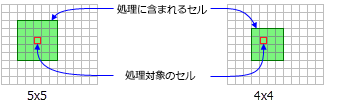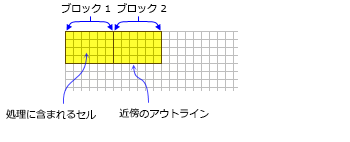# NbrRectangle

Spatial Analyst のライセンスで利用可能。

Image Analyst ライセンスで利用できます。

## サマリー

Defines a rectangle neighborhood which is created by specifying the height and the width in either map units or number of cells.

## 図NbrRectangle neighborhood examples for the FocalStatistics function.NbrRectangle neighborhood example for the BlockStatistics function (width = 6 cells, height = 4 cells).

## 説明

Tools that use neighborhood rectangle object: Block Statistics, Focal Statistics, Point Statistics, and Point Density.

##### ライセンス:

This class is also available if you have an Image Analyst extension license, but only for the Focal Statistics tool.

The rectangle neighborhood is specified by providing a width and a height in either cells or map units.

When rectangle is used in a focal tool the x, y position of the processing cell within the neighborhood is determined, with respect to the upper-left corner of the neighborhood, by the following equations:

`````` x = (width of the neighborhood + 1)/2
y = (height of the neighborhood + 1)/2``````

If the input number of cells is even, the x,y coordinates are computed using truncation.

Only the cells whose centers fall within the defined object are processed as part of the rectangle neighborhood.

## 構文

`NbrRectangle ({width}, {height}, {units})`
 パラメーター 説明 データ タイプ width The width of the rectangle neighborhood. If only the width is specified, the resulting neighborhood is a square.(デフォルト値は次のとおりです 3) Double height The height of the rectangle neighborhood. If only the height is specified, the resulting neighborhood is a square.(デフォルト値は次のとおりです 3) Double units Defines the units of the neighborhood.CELL—The unit of measurement is in cells.MAP—The units are in map coordinates.(デフォルト値は次のとおりです CELL) String

## プロパティ

 プロパティ 説明 データ タイプ width(読み書き) The width of the rectangle neighborhood. If only the width is specified, the height will default to the same as the width, resulting in a square neighborhood. Double height(読み書き) The height of the rectangle neighborhood. If only the height is specified, the width will default to the same as the height, resulting in a square neighborhood. Double units(読み書き) Defines the units of the neighborhood. String

## コードのサンプル

NbrRectangle example 1 (Python window)

Demonstrates how to create a NbrRectangle class and use it in the BlockStatistics tool within the Python window.

``````import arcpy
from arcpy import env
from arcpy.sa import *
env.workspace = "C:/sapyexamples/data"
outNbrRec = BlockStatistics("block", NbrRectangle(4, 5, "MAP"))
outNbrRec.save("C:/sapyexamples/output/blstatsnbrr2")``````
NbrRectangle example 2 (stand-alone script)

Implements the BlockStatistics tool using the NbrRectangle class.

``````# Name: NbrRectangle_Ex_02.py
# Description: Uses the NbrRectangle object to execute BlockStatistics tool.
# Requirements: Spatial Analyst Extension

# Import system modules
import arcpy
from arcpy import env
from arcpy.sa import *

# Set environment settings
env.workspace = "C:/sapyexamples/data"

# Set local variables
inRaster = "block"

# Create the Neighborhood Object
width = 5
height = 6
myNbrRec = NbrRectangle(width, height, "MAP")

# Execute BlockStatistics
outBlkStat =  BlockStatistics(inRaster, myNbrRec, "MINIMUM", "DATA")

# Save the output
outBlkStat.save("C:/sapyexamples/output/blstat_rec3")``````